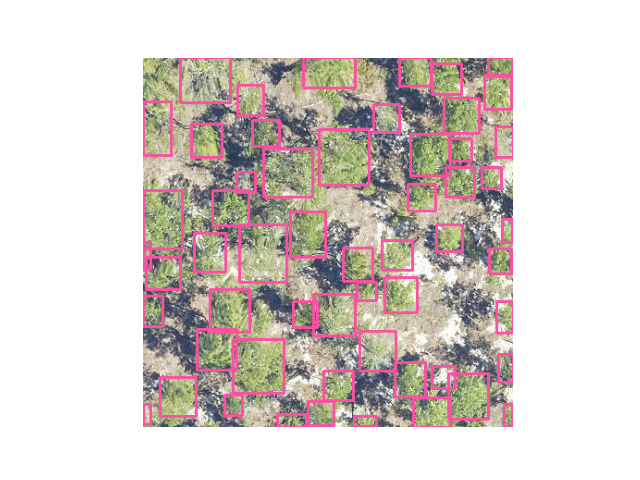# Getting started#

## How do I use a pretrained model to predict an image?#

```from deepforest import main
from deepforest import get_data
import os
import matplotlib.pyplot as plt
model = main.deepforest()
model.use_release()

img = model.predict_image(path="/Users/benweinstein/Documents/NeonTreeEvaluation/evaluation/RGB/TEAK_049_2019.tif",return_plot=True)

#predict_image returns plot in BlueGreenRed (opencv style), but matplotlib likes RedGreenBlue, switch the channel order.
plt.imshow(img[:,:,::-1])
```** please note that this video was made before the deepforest-pytorch -> deepforest name change. **

For single images, `predict_image` can read an image from memory or file and return predicted tree bounding boxes.

### Sample data#

DeepForest comes with a small set of sample data that can be used to test out the provided examples. The data resides in the DeepForest data directory. Use the `get_data` helper function to locate the path to this directory, if needed.

```sample_image = get_data("OSBS_029.png")
sample_image
'/Users/benweinstein/Documents/DeepForest/deepforest/data/OSBS_029.png'
```

To use images other than those in the sample data directory, provide the full path for the images.

```image_path = get_data("OSBS_029.png")
boxes = model.predict_image(path=image_path, return_plot = False)
```
```boxes.head()
xmin        ymin        xmax        ymax  label    scores
0  334.708405  342.333954  375.941376  392.187531      0  0.736650
1  295.990601  371.456604  331.521240  400.000000      0  0.714327
2  216.828201  207.996216  245.123276  240.167023      0  0.691064
3  276.206848  330.758636  303.309631  363.038422      0  0.690987
4  328.604736   45.947182  361.095276   80.635254      0  0.638212
```

For the release model, there is only one category “Tree”, which is numeric 0 label.

### Predict a tile#

Large tiles covering wide geographic extents cannot fit into memory during prediction and would yield poor results due to the density of bounding boxes. Often provided as geospatial .tif files, remote sensing data is best suited for the `predict_tile` function, which splits the tile into overlapping windows, performs prediction on each of the windows, and then reassembles the resulting annotations.

Let’s show an example with a small image. For larger images, patch_size should be increased.

```raster_path = get_data("OSBS_029.tif")
# Window size of 300px with an overlap of 25% among windows for this small tile.
predicted_raster = model.predict_tile(raster_path, return_plot = True, patch_size=300,patch_overlap=0.25)
```

** Please note the predict tile function is sensitive to patch_size, especially when using the prebuilt model on new data**

We encourage users to try out a variety of patch sizes. For 0.1m data, 400-800px per window is appropriate, but it will depend on the density of tree plots. For coarser resolution tiles, >800px patch sizes have been effective, but we welcome feedback from users using a variety of spatial resolutions.

### Predict a set of annotations#

During evaluation of ground truth data, it is useful to have a way to predict a set of images and combine them into a single data frame. The `predict_generator` method allows a user to point towards a file of annotations and returns the predictions for all images.

Consider a headerless annotations.csv file in the following format

```image_path, xmin, ymin, xmax, ymax, label
```

with each bounding box on a separate row. The image path is relative to the root dir. Its often easiest to just save the .csv file alongside the images.

We can view predictions by supplying a save dir (”.” = current directory). Predictions in green, annotations in black.

```csv_file = get_data("testfile_deepforest.csv")
boxes = model.predict_file(csv_file=csv_file, root_dir = os.path.dirname(csv_file),savedir=".")
```

### Projecting predictions#

DeepForest operates on image data without any concept of geopgraphic projection. Annotations and predictions are made in reference to the image coordinate system (0,0). It is often useful to transform this to geographic coordinates. Rasterio provides us with helpful utilities to do this based on a projected image.

For example, consider some sample data that comes with package. We can read in the image, get the transform and coordinate reference system (crs).

```    img = get_data("OSBS_029.tif")
r = rasterio.open(img)
transform = r.transform
crs = r.crs
print(crs)
```
```    CRS.from_epsg(32617)
```

We can predict tree crowns in the image and then convert them back into projected space

```    m = main.deepforest()
m.use_release(check_release=False)
df = m.predict_image(path=img)
gdf = utilities.annotations_to_shapefile(df, transform=transform, crs=crs)
```
```    gdf.total_bounds
array([ 404211.95, 3285102.85,  404251.95, 3285142.85])
gdf.crs
<Projected CRS: EPSG:32617>
Name: WGS 84 / UTM zone 17N
Axis Info [cartesian]:
- [east]: Easting (metre)
- [north]: Northing (metre)
Area of Use:
- undefined
Coordinate Operation:
- name: UTM zone 17N
- method: Transverse Mercator
Datum: World Geodetic System 1984
- Ellipsoid: WGS 84
- Prime Meridian: Greenwich
```

### Customizing box appearance for predictions#

The color and line thickness of boxes can be customized using the `color` and `thickness` arguments. `color` is the color of the bounding box as a tuple of BGR color, e.g. orange annotations is (0, 165, 255). `thickness` is the thickness of the rectangle border line in pixels.

```image_path = get_data("OSBS_029.png")
boxes = model.predict_image(path=image_path, return_plot = True, color=(0, 165, 255), thickness=3)
```